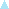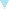# TUSNELDA Query SyntaxSFB 441Project C1Sustainable Data FormatsAnnotation standardSubmit a queryAs we use XMLQUERY as search engine for TUSNELDA, parts of the following specifications have been adopted from the XMLQUERY documentation.

## Query language

A query is an expression built up from atomic constants and operators as specified below.

## Atomic expressions

 Type Precedence Operator BRACKET 0 . . matches any XML element BRACKET 0 # # matches any piece of PCDATA BRACKET 0 quoted string a string valued constant BRACKET 0 numeric constant a numeric constant BRACKET 0 name the name of an XML element or attribute

## Operators allowed in query language

There are really two sublanguages in the query language, one which describes the tree structure of the elements you want to find, the other is a language for expressing boolean conditions on the attribute values of these elements.

### Tree structure

 Type Precedence Operator BINOPR 200 / A/B if a B is a child of an A BINOPR 100 , A,B if a B is right sibling of an A BINOPR 150 & A & B if an A and a B in any order MONPOST 400 * A* is multiple occurances of A. This is context dependant, if before a /, then this means multiple nested occurances, if not before a /, then this means multiple sequential occurances. MONPOST 400 ? A? if there is an optional occurance of A BINOPR 250 | A|B if an A or a B

### Relational operators

 Type Precedence Operator BINOPL 300 = string equality BINOPL 300 == numeric equality BINOPL 300 > greater than BINOPL 300 < less than BINOPL 300 >= greater than or equal BINOPL 300 <= less than or equal BINOPL 300 ~ string regular expression match BINOPL 300 != string inequality BINOPL 300 !== numeric inequality BINOPL 300 !~ string regular expression non-match

### Arithmetic and string operators

 Type Precedence Operator BINOPL 500 + numeric plus BINOPL 500 - numeric minus

### Other constants and operators

 Type Precedence Operator MONPRE 400 % introduces variables MONPRE 400 \$ introduces variables (as %) MONPRE 400 - numeric negation MONPOST 270 ! marking something to be saved MONPOST 400 [ ] A[B] if there is an A which satisfies boolean condition B BRACKET 0 ( ) general purpose brackets BRACKET 0 ^ Marks the beginning (i.e. before the first child) of an element BRACKET 0 \$ Marks the end (i.e. after the last child) of an element

## Query language syntax

In more detail, the query language syntax is described below. Text in green are literal operators, text in italics are nonterminals in the grammar. The grammar below is ambiguous, as usual ambiguities are resolved using the operator precedences given above (operators with higher precedence bind more tightly).

The syntax of an tree_expression is
 tree_expression :== tree_expression / tree_expression :== tree_expression & tree_expression :== tree_expression , tree_expression :== tree_expression | tree_expression :== ( tree_expression ) :== tree_expression * :== tree_expression ! :== element_expression :== ^ :== \$

The syntax of an element_expression is
 element_expression :== element_name_expression ( [ boolean_expression ] )? :== #   string_bool_op   string_expression
 element_name_expression :== . :== # :== (element_name_expression ) :== \$ variable_name :== % variable_name :== element_name :== element_name_expression | element_name_expression
 boolean_expression :== boolean_expression , boolean_expression :== boolean_expression | boolean_expression :== string_expression   string_bool_op   string_expression :== number_expression   number_bool_op   number_expression :== \$ variable_name :== % variable_name :== number_expression
 number_expression :== number_expression   arith_op   number_expression :== number_literal
 string_expression :== number_literal :== quoted_string_literal :== attribute_name :== # :== \$ variable_name :== % variable_name
 number_bool_op :== == | !== | < | > | <= | >=
 string_bool_op :== = | != | ~ | !~
 arith_op :== + | -
To be continued.

## Example queries

```   .*/sp!/.*/#~"Idefiks"
```
matches every <sp> element which contains the text string "Idefiks" (matches in the B8 Comic Corpus).
```   .*/sp!/.*/reg
```
matches every <sp> element which contains a <reg> element (matches in the B8 sub-corpora).
```   .*/sp/.*/reg
```
same as above, but does not display the matching <sp> elements (which must be indicated by the ! operator) but only the number of matches found.
```   .*/sp!/.*/reg!
```
same as above, but displays each <sp> element once for each <reg> element in it, highlighting the <reg> element.
```   .*/sp!/.*/marked[type="deic-loc"]
```
matches every <sp> element which contains a local deictic (matches in the B8 and B9 sub-corpora)
```   .*/.!/marked[type="deic-loc"]
```
matches any element which contains a local deictic as immediate child.
```   .*/figure!/.*/situation/keywords/term/#~"forefinger"
```
matches every <figure> element which contains a situational characterization with the keyword "forefinger" (matches in the B8 Comic Corpus).
```   .*/figure!/.*/situation/keywords/(term/#~"forefinger" & term/#~"bent")
```
matches every <figure> element which contains a situational characterization with the keywords "forefinger" and "bent" (matches in the B8 Comic Corpus).
```   .*/((sp/spokenpar!/ptr[target=%X]),.*,figure[id=%X]!)
```
matches every spoken paragraph which contains a pointer to a figure and the corresponding figure (matches in the B9 text Brasil - soccer match (tv).